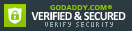ISBN: 0130869988

ISBN13: 9780130869982

# Discrete Mathematics with Combinatorics

Placing each topic in context, this text covers concepts associated with discrete mathematical systems that have applications in computer science, engineering, and mathematics. It introduces basic... This description may be from another edition of this product.

## Selected

Format: Hardcover

Condition: Acceptable

\$13.29
Save \$98.04!
List Price \$111.33

1 Available

## Customer Reviews

1 rating

### It is high on my list of potential textbooks for a course in discrete math

I occasionally teach discrete mathematics at the college level and so I am always trolling in a search for a better textbook. That is the context within which I examined this book. The coverage of the basic topics is exactly the way I prefer in both order and content. The initial chapters are: *) Truth tables, logic and proofs *) Set theory *) Logic, integers and proofs *) Functions and matrices I would never use a book that did not cover logic, set theory, relations and functions in that order. The remaining topics in the order they appear are: *) Algorithms and recursion *) Graphs, directed graphs and trees *) Number theory *) Counting and probability *) Algebraic structures *) Number theory revisited *) Recursion revisited *) Counting continued *) Generating functions *) Graphs revisited *) Trees *) Networks *) Theory of computation *) Theory of codes *) Enumeration of colors *) Rings, integral domains and fields *) Group and semigroup characters *) Applications of number theory As can be seen from this list, there is much more material here than can be covered in a one-semester course. However, the sections are independent enough that selection can be done. For example, the "revisited" chapters could be skipped. This revisiting means that the depth of coverage is there if you have need of it. A large number of exercises are included at the end of the chapters and solutions to many of them are included. I do not know if and when I will teach discrete math again. If that situation does arise again, I will give this book strong consideration as the choice of text.